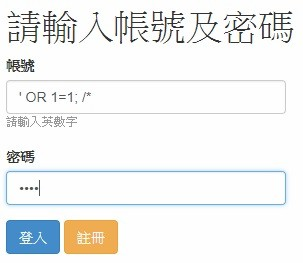#2018 iT 邦幫忙鐵人賽DAY 29
4
Modern Web

## [Day 29] 用ASP.NET MVC 做簡單的註冊及登入系統(五) - SQL Injection

SQL Injection 的多種攻擊方式與防護討論

SQL Injection簡單說就是利用程式的漏洞，原本應該是要輸入正確的帳號密碼才可以登入，但是以我昨天的語法，裡面有個很大的漏洞，語法如下

``````string strSQL = "SELECT 1 FROM `userdata` WHERE `account` = '" + account + "' AND `password` = '" + password + "';";
````````````SELECT * FROM `userdata` WHERE `account` = '' or 1=1; /* and `password` = '*/--';
````````````SELECT 1 FROM `userdata` WHERE `account` = '' OR 1=1 OR '' AND `password` = '(輸入的內容)';
``````

• `account` = '' 這個條件一定不會成立
• 1=1 這個條件一定會成立
• '' AND `password` = '(輸入的內容)' 這個條件一定不會成立

``````string strSQL = "SELECT 1 FROM `userdata` WHERE `account` = @account AND `password` @password;";
MySqlCommand cmd = new MySqlCommand(strSQL, conn);
cmd.Parameters.Add("@account", MySqlDbType.VarChar).Value = account;
cmd.Parameters.Add("@password", MySqlDbType.VarChar).Value = password;
``````

``````public bool CheckUserData(string account, string password)
{
try
{
Connect();
string strSQL = "SELECT 1 FROM `userdata` WHERE `account` = @account AND `password` = @password;";
MySqlCommand cmd = new MySqlCommand(strSQL, conn);
cmd.Parameters.Add("@account", MySqlDbType.VarChar).Value = account;
cmd.Parameters.Add("@password", MySqlDbType.VarChar).Value = password;

using (MySqlDataReader reader = cmd.ExecuteReader())
{
if (reader.Read())
return true;
}
return false;
}
catch(Exception ex)
{
string error = ex.ToString();
return false;
}
finally
{
Disconnect();
}
}
``````--